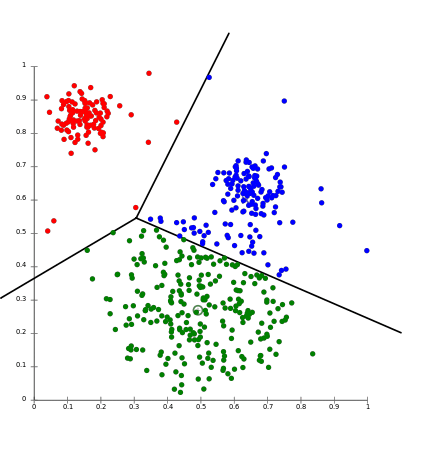# Model Performance Callibration

## Accuracy

When an algorithm gives out probability scores accuracy is not a good measure. Accuray does not have a clean way to in-corporate probability scores.

The above table shows a toy test dataset . We also have two models Model1 and Model2. Two data points have the true class label 1 and the other have 0. The next two columns display the probability of y = 1 that is p(yq=1).

Y1hat and Y2hat are the predicted class labels for the points.

What is the inference from this result? Let’s first discuss the probability scores of model 1.This model predicts that two points belong to class 1 with a high probability score of 0.72 and 0.65 and the other two points with class label 0 with probability scores of 0.28 and 0.35. In the same breadth when we discuss Model 2 we find that this model is performing slightly lower as the probability scores are 0.6 and 0.55 for class label 1. For class label 0 the scores are 0.4 and 0.45 so the model is not doing a good job since the scores are less than 0.5. Finally we would recommend Model 1 as the sores are good when compared to Model 2.

If we have to report the acuracy of these models then we would take into account not the probability scores but the counts of predicted class labels from these models. As accuracy is the ratio of correctly classified to the total number of points then both the models will perform at par.

The user is at a dilemma as to which model to use.

Thus accuracy as a performance measure may be simple but may not be very reliable.

When models give out probability scores we will use another performance measure called LOGLOSS, which we will discuss later.

## Confusion Matrix

This metric can be used for those models that perform Binary (1 or 0) or Multi-class(we can have n-number of classes) classification.

Let’s start with binary classification task(1 or 0). As the word says we will build a matrix or a grid of 2 x 2

If the model is sensible or performs reasonably well then a & d values will be large and b &c values will be small.

## Sample

In this example TPR and TNR are high whereas FPR and FNR are low.

Which implies that the model is performing extermely well.

Also we are able to compare the +ve/-ve class metrics eventhough the dataset is im-balanced.

## Percision, Recall and F1-Score

What is Precision ?

Of all the points the model declared/predicted to be positive , what is the percentage of them are actually positive.

Precision is not worried about the -ve class. From the above formula we find that precision bothers only about positive class and not about the negative class

Examples : This being a ratio the numerator is the total number of correct postive cases that we predicted . The denominator is the total number of correct positive cases that we predicted plus the in-correct positive cases we predicted.

What is Recall ?

Of all the points that belong to the positive class, how many of them is predicted to the positive class

Example : This is just the True Positive Rate or TPR. This is again ratio where the numerator is the total number of correct positive cases predicted. The denominator is the total number of actual positive points in the dataset. From a confusion matrix perpective, this will be the sum of correct true positives (TP) and the number of points which were actually positive but the model thinks it is negative which is False Negatives(FN).

Precision is about the predicted postives whereas recall is about the acutal postives in the given training dataset. Another fact here is that these two talk only about the positive and don’t care about the negative class. In other words, we are measuring how well we are performing on the postive class only.Both Precision and Recall both have to be high and both lie between 0 and 1.

## F1-Score

If F1score is high then Precision and Recall also have to be high.

F1Score takes value between 0 and 1 , where 1 is good and 0 is bad.

Since this is a composite measurement it is not all that interpretable like when we talk about Precision it is about the predicted positives and Recall is about the actual positives in the dataset.

## LogLoss

This is a loss function .

Properties of LogLoss

• Can be used for Binary / Multi-classification
• This metric uses the probability score actually
• The value lies between 0 and infinity
• The best value is 0 coz we want all losses to be small
• This metric is hard to interpret if it has a values greater than zero
• Penalizes for small deviations is the metric
• In other words this is average negative log(probability of positive class label)

Formulae for logloss

Let’s take datapoint x1 with a probability score of 0.8 that it belongs to class label 1. This being actually a positive point and its probability score is also close to 1 the logloss value is also small and closer to zero. Datapoint x2 with probability score of 0.7 also belongs to class label 1. In x2’s case the probability score is faraway from 1 and hence the logloss value is high also far-away from zero.

For the negative class or for class label 0 the predicted probability score should be 0(since we are genrating p(y=1)). In both the above cases we find that the probability for class label should be close to 0 whereas the values are distant away from 0 . Due, to this the logloss value is higher. x3 is considerably farther , when compared to x4 thus, its logloss(0.2218))is higher when comapred to x3(0.1549).

## Receiver Operating Characteristics==> Area Under the Curve(AUC)

Used only in Binary Classification.

Let’s assume we have a model that does binary classification. This model produces two outputs one is a score(could be probability scores it can produce any other score) and also yhat the output label. If the score is high the chance the predicted class label is 1 is also high.

Data has been ordered in the descending order of the score.

We will use the concept of thresholding to generate the predicted class labels. in our case lets say tau is 0.93. Data points having score equal or above this will be given 1 and the rest of the points will have predicted label as zero as shown below.

We can either draw a confusion matrix or try to calculate the Total Positive Rate and False Positive Rate. Let’s repeat the above process for another tau value of 0.90

We can define multiple tau values and generate the predicted labels. Suppose we define m-tau vaues we will ge m-sets of predicted labels. From these m-sets we calculate m-paris of TRP and FPR.

Finally, we will plot a graph with FPR on the X-axis ad TPR on the Y-axis.

Properties of AUC

• Even for im-balanced datasets a simple or dumb model can produce high scores of AUC
• AUC didn’t care about the Yihat scores as such but only about the ordering. From the table below , we see that the sorted order of yi didn’t change even after the scores from Model 2 were tabulated. The AUC(Model 1) will be equal to the AUC(Model 2)

AUC of a random model has to be atleast 0.5. AUC of any goor or sensible model has to be above 0.5. It’s the third case that we need to worry about where the AUC of the model is less than 0.5. In this case what we do is to report 1-AUC as the AUC score and invert the predicted yihat from 0 to 1 or vice cersa. The figure below depicts a model whose AUC is less than 0.5

All the above metris will be used for classification algorithms, now we will look at the metrics used by the grand daddy of all these algorithms that is REGRESSION.

R-Squared or Co-Efficient of Determination

This is a composite metric that uses Sum of Squares of residue and Sum of Squares Total.

Properties

• Values lie between 0 and 1
• 1 is a good value
• A value of 0 for R-square implies that the model is same as simple mean model
• If R-square value is negative then the model’s performance is a worse

Case 1 SS-residual = 0

Ss-residual will be zero when the predicted yihat is same as the point for all points. Now, R-square will be 1 which is the best value.

Case 2 SS-residual < SS-total

The fraction SS-residual / Ss-total will be less than 1, giving R-square values between 1 and 0

Case 3 SS-residual = SS-total

This occurs when all the predicted yihats equals the average of the input data points. This makes R-Square equal to 0 and the Model is now the simple linear model

Case 4 SS-residual > SS-total

In this case SS-residual /SS-total is greater than 1, which will make R-sqaure negative which implies that the model is wost.

Median is a robust measure of mean the same way Median Absolute Deviation(MAD) is a robust measure of Standard Deviation.

If median is small and MAD is also small then the developed Model is good.

We can use any measure of central tendency like mean, median , std. deviation or MAD.

Mean and Std. Deviation are not robust to outliers

Median and MAD are robust to outliers.

--

--

--

## More from Janardhanan a r

In the making Machine Learner programmer music lover

Love podcasts or audiobooks? Learn on the go with our new app.

## Online Customer Buying Behavior using Sequence Embedding: A Case Study Approach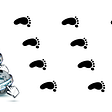## How to Overfit Your Model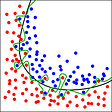## Introducing Alpa: A Compiler Architecture for Automated Model-Parallel Distributed Training That…## Crafting a Machine Learning Model to Predict Student Retention Using R## What should you do if all predictions are 0?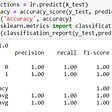## Scratch to SOTA: Build Famous Classification Nets 1 (Evaluation)## Concise Critical Review of Deep Mind’s Paper — Gato(A Generalist Agent)## Appearance-Based Gaze Estimation in the Wild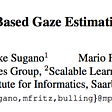## Janardhanan a r

In the making Machine Learner programmer music lover

## Are We Not entertained!?## Data-centric approach## AutoML for DSP pipeline optimization## K-Means vs. K-Medoids: An Overview